# Color Your World: More with Maps, Graphs, and Polygons

Today, guest blogger Matt Tearle continues his investigation of the new polyshape type (added in R2017b) as a mapping tool.

### Contents

#### Where were we?

In a previous post, I used the new polyshape variable type in MATLAB to determine connections between states. You can see the code in that post. I'll just load in the polygons and matrix of connections that came from that:

load stateborders

% Make a graph of state connections
G = graph(border,stnames,'lower');
% Obtain centroid locations for states
[x,y] = centroid(p);
% Plot map and connections
plot(p)
hold on
plot(G,'XData',x,'YData',y)
hold off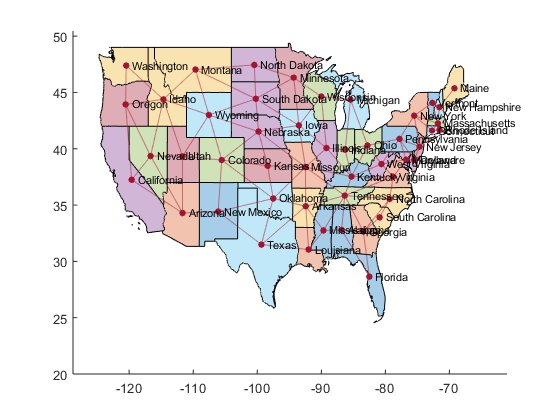Having made this nice visualization, it struck me that the colors from the default polygon plot weren't making a good map because neighboring states were often given the same color. The plot function, of course, doesn't know about the connections - it is just using a set of colors in the order of the polygons in the array. Using knowledge of the connections between states (stored in the matrix border and the graph G), I should be able to recolor the states so that no neighboring states have the same color.

#### Start simple: a greedy coloring algorithm

Note that the actual color is arbitrary. The goal is actually to assign a number to each state. That number can then be used to index into a list of colors (or a colormap).

A simple ("greedy") algorithm to assign colors would be:

1. state 1 is color 1
2. go through the states in order
3. for each state, find all the neighboring states
4. get the color values assigned to the neighboring states
5. assign the current state the smallest unused value

In the last step, if colors 1 through n are used by neighbors, then the number of colors used in the map will grow to n+1. This means that the number of colors used for the whole map will depend on the connections and the order of the states. Let's see what happens if I apply this algorithm to the states in default (alphabetical) order.

n = length(p);
color = zeros(n,1); % Initialize array to hold color numbering
numcolors = 1; % Number of colors needed (so far)
for k = 1:n
idx = neighbors(G,k); % Get neighbors of the kth node
idx = idx(idx < k);   % But just those that have an assigned color
neighborcolors = unique(color(idx)); % Get colors used by neighbors
% Assign the smallest color value not used by the neighboring nodes
thiscolor = min(setdiff(1:numcolors,neighborcolors));
% If there isn't one, add another color to the map
if isempty(thiscolor)
numcolors = numcolors + 1;
thiscolor = numcolors;
end
color(k) = thiscolor;
end
disp([num2str(numcolors),' colors needed'])

5 colors needed


Five colors is actually common for many maps.

polygonplot(p,color)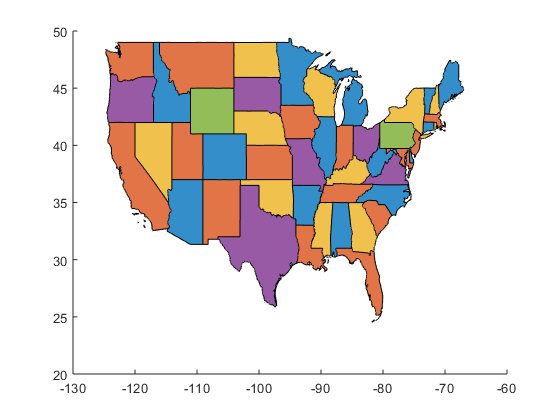I'll be plotting the states with a custom color ordering a few times, so I figured it would make sense to make a short function to do that:

type('polygonplot')

function polygonplot(p,color)
% Get a list of colors (using the default plot colors)
ax = gca;
colors = ax.ColorOrder;
% Plot the polygons and change their colors
map = plot(p);
for k = 1:length(p)
map(k).FaceColor = colors(color(k),:);
map(k).FaceAlpha = 0.8; % Make the shading darker
end


#### Greed is good?

Five colors is pretty good, but the four color mapping theorem states that you need only four colors to color any map so that no neighboring regions have the same color. However, in true pure math style, the theorem only says it can be done, not how to do it.

Is there an algorithm I can use to assign colors to the states to achieve a four-color map coloring? It turns out that this is a difficult problem in general. There are algorithms, but they are far more complicated than I want to deal with!

So instead, what about just shuffling the order of the states, then applying the greedy algorithm above? I took that code and turned it into a function that takes a graph as input and returns the color numbering and number of colors needed (equivalent to the max of the color numbering) as output.

Now I just need to shuffle the states and apply the function. But first, the matrix of connections is currently just the lower-triangular part. That structure will be ruined by the shuffling, so I'll make the full matrix version:

border = border + border';


Now let's try shuffling and see how many colors we need:

rng(123)  % for reproducibility
idx = randperm(48);
Gperm = graph(border(idx,idx));
[~,nc] = greedycolor(Gperm)

nc =
6


OK, it's working, but that particular shuffle didn't help. It actually was worse. Well, that's random numbers for you. Maybe it's a good idea to see how the number of colors needed are distributed. Let's run the algorithm a bunch of times and see:

rng(123)
nexp = 1000;
numcolors = zeros(nexp,1);
for k = 1:nexp
idx = randperm(n);
Gperm = graph(border(idx,idx));
[~,numcolors(k)] = greedycolor(Gperm);
end
histogram(numcolors)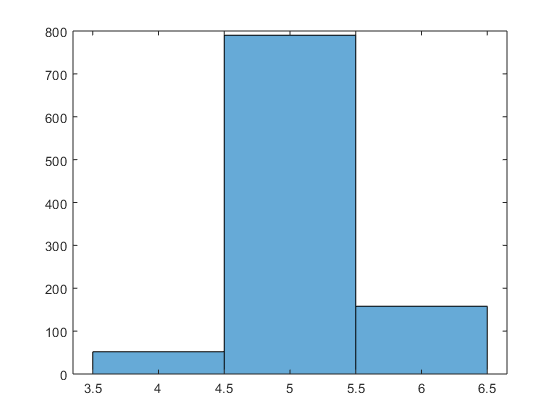Interesting. Most of the time I get five, sometimes I need six, and about 5% of the time I do indeed get a valid 4-color mapping. So a simple way to get a 4-color mapping would be to try random permutations until only four colors were needed. A terrible algorithm? Perhaps. But I bet it works:

numcolors = Inf;
while numcolors > 4
idx = randperm(n);
Gperm = graph(border(idx,idx));
[color,numcolors] = greedycolor(Gperm);
end
polygonplot(p(idx),color)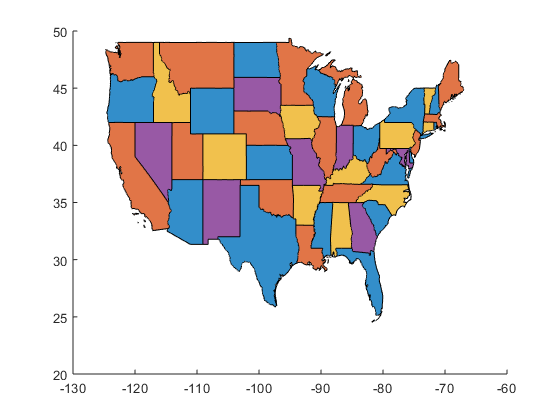#### Adding a little intelligence (but with no guarantees)

Surely there's a better way, though. Right...? Well, maybe. I did encounter an algorithm for generating 5-color mappings. Basically, remove nodes from the graph one by one, until the nodes of the remaining graph all have degree greater than five. Then... do more stuff, which will color the remaining graph. Then you add the nodes back, coloring them as you go. I didn't really pay attention to the details because it seemed like there was a very good chance that you'd never get nodes with degree greater than five. Every time you remove a node, the degree of every node it's attached to goes down by one. So it seems like you could just try to repeatedly remove the lowest-degree node. Keep the list of nodes in order that you removed them, then use that (or, more precisely, the reverse of it, from last to first) as the ordering in which to color the nodes. It's not guaranteed to work, but it's probably not a bad option to try (and surely more efficient than random permutations). Also, this was for 5-color mappings, not 4, but let's just see what we get...

G = graph(border,stnames);
idx = zeros(n,1);
for j = 1:n
[~,k] = min(degree(G));
idx(j) = find(strcmp(G.Nodes.Name{k},stnames));
G = rmnode(G,k);
end
idx = flipud(idx);
Gperm = graph(border(idx,idx));
[color,numcolors] = greedycolor(Gperm);
polygonplot(p(idx),color)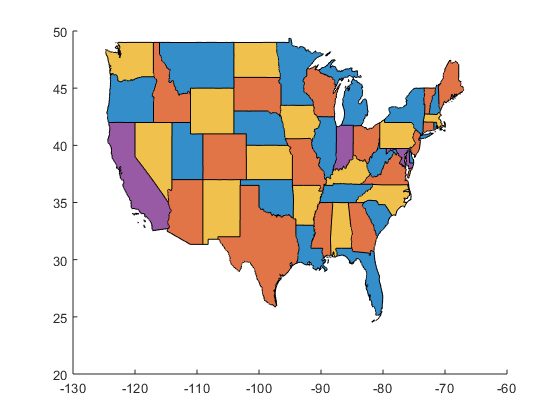And just like that, I have a 4-color mapping for the US! In fact, it's not far from being a 3-color mapping! Only three states needed the fourth color. In fact, notice that this approach tries to use the lowest numbers as much as possible

histogram(color)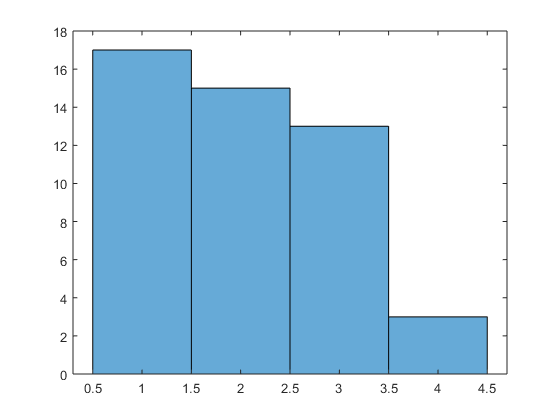But maybe I just got lucky. So let's try this on another map. Feeling brave, I found a world map shape file online. I applied the exact same approach: imported it with shaperead, converted the resulting structure array to a polygon array, used the "pad-and-intersect" method to build the graph of shared borders, sorted by iteratively removing the lowest-degree node, then applied the greedy coloring algorithm. The result...?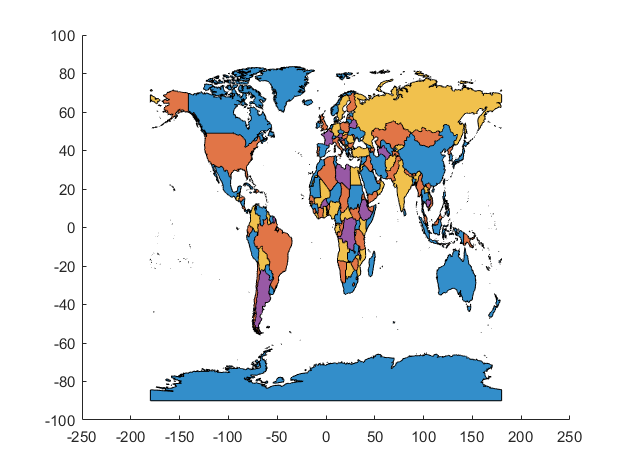A 4-color world map! Just like that.

#### How far can this go?

As I undertand it, real cartographers wouldn't have much use for my algorithm. There's a definite skew in the colors (e.g., all islands are color #1 because they have no borders), so the result isn't particularly aesthetically pleasing. Are there any amateur (or professional!) cartographers out there who want to try applying a "real" map-coloring algorithm using polygons and graphs? I'd love to see your results. I also know there must be maps that break my algorithm, but how well will the algorithm hold up with actual maps in practice, rather than deliberately pathological examples? If anyone has other polygonal maps they want to try coloring with this approach, I'd love to hear how it works.

And, of course, maps and graphs don't have to be just about physical geography - they can be abstractions of any number of things. If you have an application that could use map coloring for visualization, share your ideas in the comments.

Published with MATLAB® R2017b

|